# Base 60: Digits and terminology

by Jakub Marian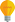Tip: See my list of the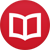Most Common Mistakes in English. It will teach you how to avoid mis­takes with com­mas, pre­pos­i­tions, ir­reg­u­lar verbs, and much more.

Ideally, every number (in its abstractness) should have its own symbol. However, since there are infinitely many natural numbers and our minds are limited, various methods to denote large numbers using only a finite set of symbols have been developed. Because of its usefulness in applications, the positional number system (which we use today) was at last adopted by many civilizations, including the modern “western” civilization. What differed among civilizations was the base – the standard base now is 10, but the Maya civilization, for example, used the base 20, and some native-American tribes used a base-4 system.

Probably the most interesting base which humans are able to use in practice is 60. In fact, it is used even today (in principle), although its numbers are written using base 10. For example, we have 60 seconds in a minute, 60 minutes in an hour, 360 degrees (a multiple of 60) to measure angles, etc.

People found the number 60 so important that in many languages it has its own name (apart from “five dozen”). What’s so special about this number? It’s all about its number of divisors. 60 can be divided by 2, 3, 4, 5, 6, 12, 10, 12, 15, 20, 30, and, of course, 60. This makes it in fact a better candidate for the base of the “percent” notation, because we commonly use thirds and sixths and 60 divided by 3 or 6 is an integer. The more divisors the base of a positional system has, the more conveniently division and multiplication can be performed in the system.

Old Babylonians are probably the only people known to have used base 60 as their primary counting system. However, their digits (the actual symbols) were very large and hence unusable in practice.

My goal was to create a new digit system in which the digits would be suitable for practical purposes and would manifest some sort of mathematical logic. Here’s what I came up with: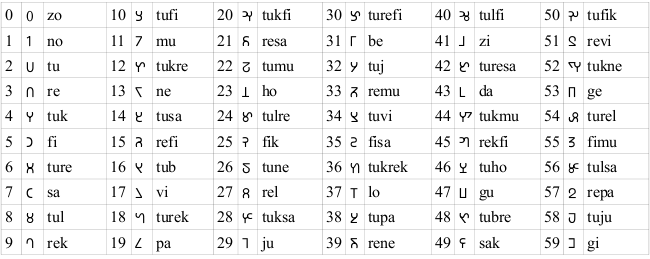The principle is simple (and described in detail below): Symbols of prime numbers don’t contain any “middle” part and all composite numbers contain all symbols for the primes present in their prime decomposition. The vertical line, circle and slanted lines denote the second to the fifth power (of the prime they are attached to).

You can also download a font containing all the symbols. If you use it publicly, I kindly ask you to include a link back to this article. The numbers 0–60 are represented by the characters 0–9, a–z, and A–Y.

## Symbols of prime numbers

I divide the prime numbers into five groups (to remember them more easily). It is convenient to represent the numbers visually to be able to count in base 60 without having to use base 10. A good way is to imagine dots in plane. That’s why I list the numbers together with a “decomposition” as a sum of a square and a small number. The syllable next to the symbol is the name of a prime.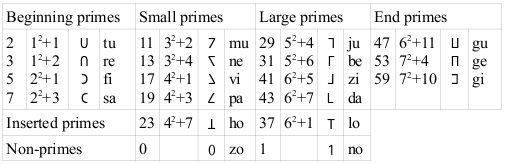Small and large primes share many similarities. They are all twin primes and the symbols of their twins can be found by reflecting their symbols horizontally. In fact, all twin primes share this logic (not only those in the two mentioned groups) and all primes that don’t have a twin have a horizontally symmetric symbol.

Inserted primes are “dual” in the sense that their sum is 60. Beginning and end primes also share many similarities. Tu and re, as well as gu and ge aren’t twin primes (and hence their symbol is symmetric). The symbol for gi suggests that it has its twin – and indeed it has, but 61 is not a digit.

## Names of primes and pronunciation

The structure of names of primes follows the division into groups described in the previous section. The ending vowels of the primes in 4-member groups are -u, -e, -i, -a. Inserted primes and non primes end with the vowel -o. The consonants are chosen in such way, so that all primes are easily distinguishable from each other. Thus, they are different for all primes, apart from the rarely used end primes for which “g” symbolizes that they are big (great, grand, giga, giant…).

Powers of primes are determined by suffixes: -k for the second, -l for the third, -b for the fourth and -j for the fifth (and they are chosen so that there’s no possibility for a letter to appear twice in a row when creating longer names of numbers). So, for example, rek = 9 (re = 3, -k = 2), tub = 16 (tu = 2, -b = 4). Powers are denoted by adding a vertical line for the second power, a circle for the third, and slanted lines for the fourth and fifth as you will see in the table of all numbers.

The pronunciation is completely regular. “g” is always pronounced as in “get”. Vowels are always pronounced as in “put”, “get”, “fit”, “car” and “hot”. So, for example, “be”, as a number, is pronounced as the first two letters in “bed”, “fi” as the first two letters in “fit” and “no” as the first two letters in “not”.

## Composite numbers

Symbols for composite numbers contain all symbols of its prime components with an appropriate symbol for their power. The symbols are not created completely regularly, but if you follow the rule that the power of a prime is always (with an exception of rel) at the bottom of its symbol, you will find there’s usually only one natural way to put the symbols into a contiguous larger symbol. All numbers from 0 to 60 are:If we want follow the traditional way to call larger numbers, that is “digit order digit order” (as in “three thousand seven hundred”), we have to create names also for the orders, that is, 60, 3600, etc. (the equivalent of 10, 100, etc. in our new base). I’ve chosen the following names:All consonants use different manners of articulation (nasal, fricative, sibilant, voiceless plosive, and voiced plosive) and the position of articulation is order from front to back (bilabial, labiodental, alveolar and velar). They also don’t collide with the letter denoting powers of primes. The letter “y” in is pronounced the same way as in the English word “my”.

Numbers above 60 follow completely regularly the form “digit order digit order…”. If the first digit is 1 or the last digit is 0, it can be dropped, so, for example, myno, mytu and myre is sixty one, sixty two and sixty three. Tumy is one hundred twenty, tumyse is one hundred twenty seven etc. (for example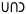= tufyremyfi = seven thousand three hundred eighty five).

By following this algorithm we created names for numbers up to gigygitygisygifygimygi = 46 655 999 999 = an utterly long name in English. Then, we use the following notation: A-B = A x 606 + B, so, for example, no-zo is “(no times 606) + 0”, i.e. 606. The largest number having its own name is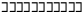= gigygitygisygifygimygi-gigygitygisygifygimygi = 36 279 705 599 999 999 999 = thirty six quintillion two hundred seventy nine quadrillion seven hundred five trillion five hundred ninety nine billion nine hundred ninety nine million nine hundred ninety nine thousand nine hundred ninety nine.

By the way, I have written several educational ebooks. If you get a copy, you can learn new things and support this website at the same time—why don’t you check them out?